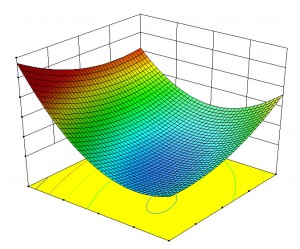## Applied Statistics FOR ENGINEERS AND SCIENTISTS – LIVE ONLINEIn this course you will learn the fundamental concepts of applied statistics including descriptive statistics, confidence intervals, hypothesis tests, regression analysis, and analysis of variance, and be able to apply them immediately to the problems that you encounter on the job. This will be accomplished by lectures that carefully explain each statistical technique and then illustrated by one or more examples using real-world data. This is reinforced by an extensive number of in-class exercises that students perform using Excel. Whether you are new to statistics or looking for a refresher course, you will find this live online seminar (using Zoom) a great way to get up to speed in just four days.  The instructor, Dr. Averill M. Law, will be available after class to answer your individual questions.

Versions of this seminar have been presented to Caterpillar, Institute of Industrial and Systems Engineers (IISE), Lockheed Martin, the U.S. Army, and the U.S. Navy (four times).

## Course Schedule

Course Dates Location Cost Cost with early registration discount
Applied Statistics for Engineers and Scientists TBA Live Online with Zoom \$2200

## Outline

### What You Will Learn (look to the right for more detail):

1. Populations and Samples
2. Random Variables and Probability Distributions
3. Point Estimation
4. Descriptive Statistics
5. Confidence Intervals Based on a Single Sample
6. Hypothesis Tests Based on a Single Sample
7. Inferences Based on Two Samples
8. Regression Analysis
9. Analysis of Variance (ANOVA)

### Critical Questions That the Seminar Will Answer:

• How do you summarize and describe your data using descriptive statistics and plots to determine its center, spread, and symmetry?
• How do you estimate the mean or a proportion corresponding to a single data set and determine its precision (confidence intervals)?
• How do you test whether the mean or a proportion is equal to a hypothesized value for a single data set (hypothesis tests)?
• How do you compare the means or proportions for two different data sets?
• How do you develop and validate a probabilistic model that relates a dependent variable to one or more independent variables, which can then be used for prediction or description (regression analysis)?
• How do you test whether the means or probability distributions corresponding to three or more different data sets are equal (analysis of variance)?
• How do you proceed when the assumptions (e.g., normally distributed data) for a statistical procedure are not satisfied in practice?